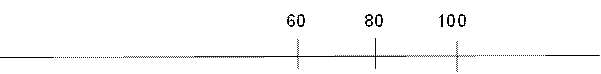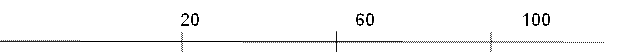# CAT 2021 Question Paper | Quant Slot 1

###### CAT Previous Year Paper | CAT Quant Questions | Question 2

CAT 2021 Quant was dominated by Arithmetic followed by Algebra. In Arithmetic, the questions were dominated by topics like Speed-time-distance, Mixture and Alligations. This year, there was a surprise. The questions from Geometry were relatively on the lower side as compared to the previous years. There were 8 TITA Qs this year. Overall this section was at a medium level of difficulty.

Question 2 : The number of integers n that satisfy the inequalities |n - 60| < |n - 100| < |n - 20| is

1. 21
2. 18
3. 20
4. 19

## Best CAT Coaching in Chennai

#### CAT Coaching in Chennai - CAT 2022Limited Seats Available - Register Now!

|n - 60| < |n - 100| < |n - 20|
To solve this question, we need to know the basic concept that ‘if x and y are two points on the number line, then the distance between these two points can be represented by |x - y| or |y - x|.’
Let's take the first part of the inequality
|n - 60| < |n - 100|This inequality holds good for the values of n below 80. So this is the turning point.
Hence ‘n’ should be less than 80.
Now Let's check for the later part of the inequality.
|n - 100| < |n - 20|This inequality holds good for the values of n above 60. So, 60 is the turning point here.
Hence ‘n’ should be greater than 60.
Therefore, the values of ‘n’ range from 61 to 79.
So, the total possible integers that satisfy this inequality are 19.

The question is " The number of integers n that satisfy the inequalities |n - 60| < |n - 100| < |n - 20| is "

##### Hence, the answer is '19'

Choice D is the correct answer.

###### CAT Coaching in ChennaiCAT 2022

Classroom Batches Starting Now! @Gopalapuram

###### Best CAT Coaching in Chennai Introductory offer of 5000/-

Attend a Demo Class

##### Where is 2IIM located?

2IIM Online CAT Coaching
A Fermat Education Initiative,
58/16, Indira Gandhi Street,
Kaveri Rangan Nagar, Saligramam, Chennai 600 093

##### How to reach 2IIM?

Phone: (91) 44 4505 8484
Mobile: (91) 99626 48484 / 94459 38484
WhatsApp: WhatsApp Now
Email: info@2iim.com